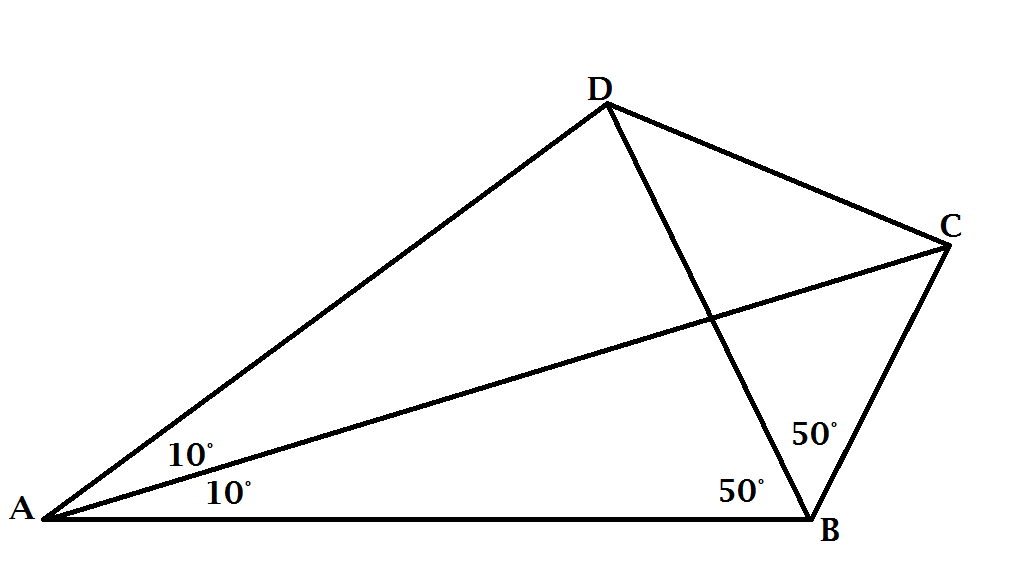# Find the Angle #2$\angle CAD = \angle BAC = 10^\circ$ and $\angle DBA = \angle CBD = 50^\circ$.

Find $\angle BDC$.

×